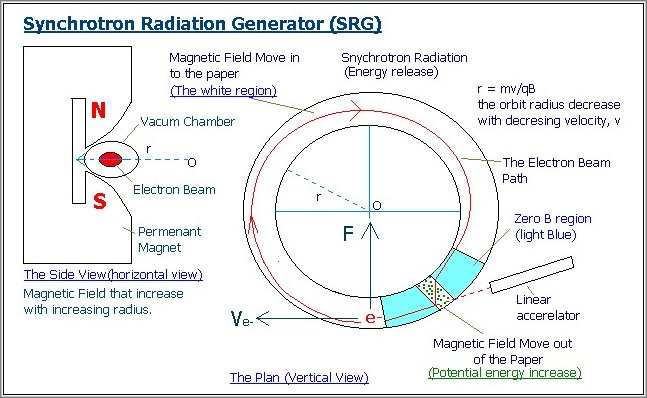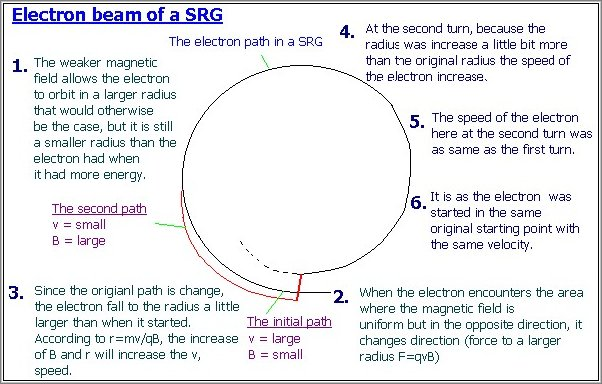• 1. When the speed of the electron beam decrease, the radius of the electron orbit also decrease (a few percent) according to this formula: r = mv/qB.

• 2. When a electron is force in to a weaker magnetic field (B) region, the velocity decrease. Yet, in this SRG, the electron beam (the charge particle) only enter the weaker B region after it HAD ALREADY slow down.(please remember that the SRG magnetic field sharply increases with increasing radius).

• 3. Then, the electron beam is force in to a stronger magnetic field region

• 4. As a result, the entering to an increase magnetic field region increases the velocities of electrons.

## And now the whole article: Abstract: Synchrotron Radiation Generator (SRG):

The SRG consists of an evacuated tube that is formed into a circular loop and embedded in an permanent magnet in which the magnetic field is in the vertical direction (the electron orbital plane is horizontal). It is very much as same as a common cyclic accelerator only without any external electric field. In this version of SRG, the poles of the magnet are tapered to cause the field near the orbit to weaken with decreasing radius to keep the particles on the difference radius trajectory, inside the vacuum chamber. In other word, as the electron in a SRG are accelerated, the strength of the magnetic field IS NOT increased to keep the radius of the orbit approximately constant.After emission from the cathode and after acceleration to a fixed velocity, the electrons are injected into the evacuated tube and it accelerates in the tube. The path of the particle inside the tube is therefore circular. The electrons moving in curved paths radiate electromagnetic energy. The intensity of this radiation is commonly called synchrotron radiation and these radiation carry energy away from the electron. With less energy, the electron slows down.When the speed of the electron beam decrease, the radius of the electron orbit also decrease (a few percent) according to this formula: r = mv/qB. Otherwise, as in a common betatron where the guiding magnetic field has a fixed intensity, the electron beam slows down, the radius will decrease faster, and it will hit the inner wall faster too.

When a electron is force in to a weaker magnetic field (B) region, the velocity decrease. Yet, in this SRG, the electron beam (the charge particle) only enter the weaker B region after it HAD ALREADY slow down. Meanwhile, if the electron in the common particle accelerator, accelerate (after gaining energy) to a stronger B region (r=mv/qB), the speed would not increase by the mean of particles are accelerated by increasing the magnetic field as in a betatron.

The strong fields keep the energy loss large by guiding the electrons on hardly curved paths

When the electron almost makes a complete orbit, the electron beam enters a second region at the cyclic chamber where the magnetic field is nearly uniform, but in the opposite direction to the first magnetic field. The field will there for exert a force on the opposite direction of the original one and thus increasing the first electron orbit radius.

Then, after passing through the tiny second magnetic field region, the electron beam is once again guided back to it first circular path with it orbit radius increase starting the cycle all over again. Thus, the electron beam is force in to a stronger magnetic field region (please remember that the SRG magnetic field sharply increases with increasing radius).

As a result, the entering to an increase magnetic field region increases the velocities of electrons. The synchrotron radiation energy losses during the first cycle are carefully compensated.

Thank you a lot for spending your time reading this short message.

Original Author -----

April 16, 2001